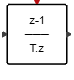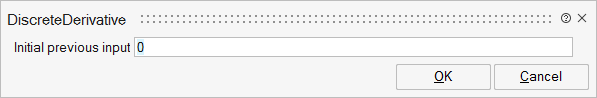# DiscreteDerivative

This block computes the discrete derivative of the input signal. The derivative is obtained by dividing the difference between the current value of the input and the previous value of the input divided by the difference of their respective times.Dynamical

## Description

The DiscreteDerivative computes the discrete derivative of the input signal. The derivative is obtained by dividing the difference between the current value of the input and the previous value of the input divided by the difference of their respective times. If the time difference is zero, the block outputs the previous value of the derivative. The value of the input at the initial time is defined by the block parameter. For initialization, the block parameter sets the value of the initial previous input. This block supports double data type.

## ParametersNameLabelDescriptionData TypeValid Values

init_cond

Initial previous input

## Ports

NameTypeDescriptionIO TypeNumber

Port 1

explicit

input

1

Port 2

explicit

output

1

Port 3

activation

input

1